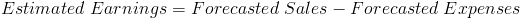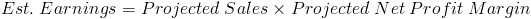# Estimated Earnings

Estimated Earnings Calculator (Click Here or Scroll Down)The formula for estimated earnings is forecasted sales minus forecasted expenses. The formula above is a simple way of restating how to calculate net income, i.e. earnings, based on its estimated components. However, the practice of calculating estimated earnings is far more complex.

It is important to note that the expenses in the estimated earnings formula should include interest and taxes.

## Estimated Earnings with Profit Margin Formula

Another simple way to state the estimated earnings formula isThis formula of projected sales times the projected net profit margin can be used because net profit margin is net income divided by sales. By multiplying net sales and net profit margin, sales will be canceled out which leaves net income(earnings). Again, this is a general concept but calculating the actual estimates are more complex.

## Calculating Estimated Earnings

Internally, a company may calculate estimated earnings for a particular project or company changes with pro forma income statements. Any changes of a company's operations should be taken into consideration when traversing from what earnings is known today to what earnings is estimated to come, and pro forma statements can provide this information.

Estimated earnings can also be calculated by trends. By looking at prior trends, regression analysis can be used to determine an estimate of earnings.

Scenario analysis can also be used as a method to calculate estimated earnings. Scenario analysis looks at different situations that may occur, ranging from the worst case scenario to the best case scenario to avoid analyzing in broad strokes. The state of the entire economy should be factored into estimate calculations. Scenario analysis can include many factors including giving weight to the overall economy.

There are various ways to calculate the variables of the estimated earnings formula. The different methods can also be used in combination to narrow any outlier estimates that vary dramatically and don't paint a true picture of what earnings will be.

New to Finance?